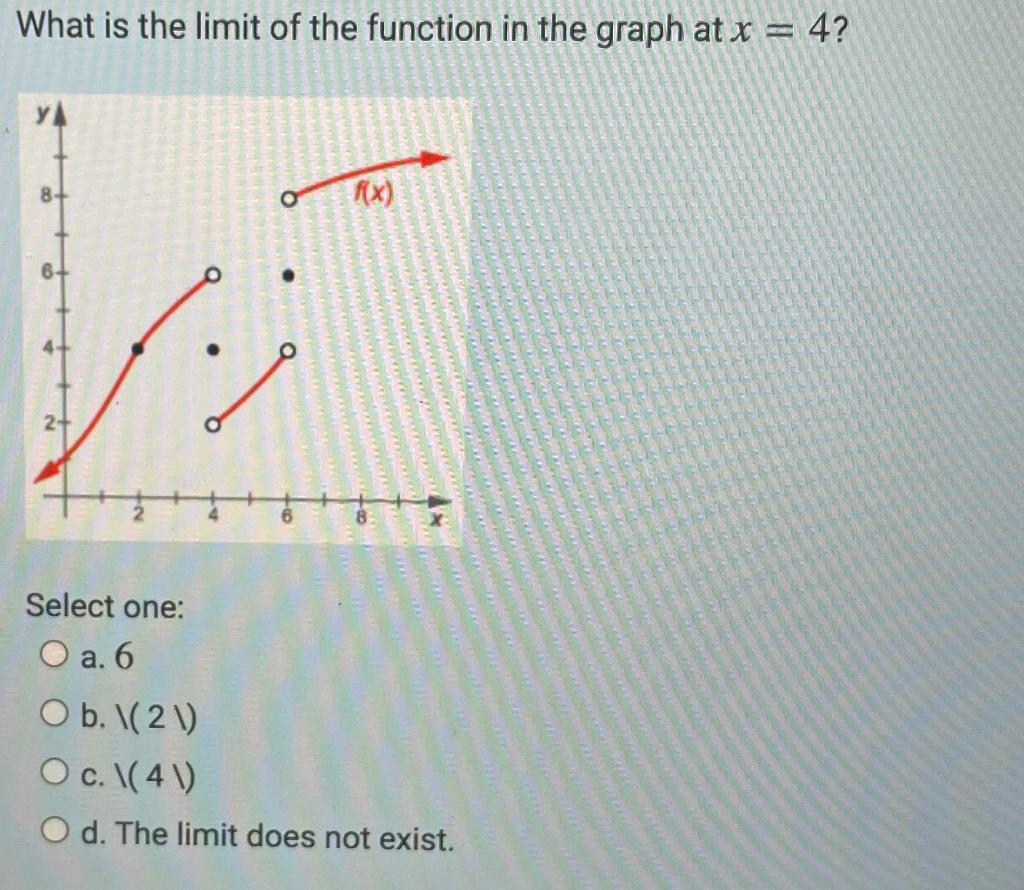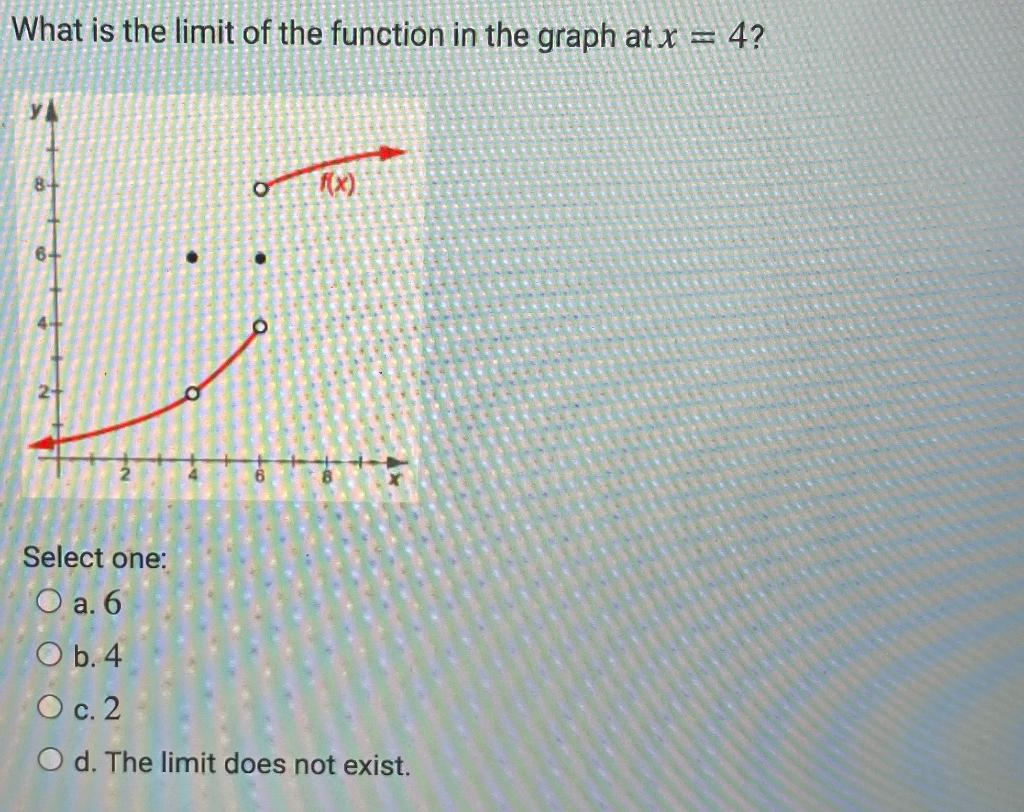### Create an Account

Home / Questions / What is the limit of the function in the graph at x = 4? YA f(x) 6- O 2+ Select one: O a. ...

# What is the limit of the function in the graph at x = 4? YA f(x) 6- O 2+ Select one: O a. 6 O b. (21) O c. 1(41) O d. The limit does not exist. What is the limit of the function in the graph at x = 4?

What is the limit of the function in the graph at x = 4? YA f(x) 6- O 2+ Select one: O a. 6 O b. (21) O c. 1(41) O d. The limit does not exist.
What is the limit of the function in the graph at x = 4? 8 O f(x) X Select one: O a. 6 O b.4 O c. 2 O d. The limit does not exist.May 05 2021 View more View LessSubscribe To Get Solution